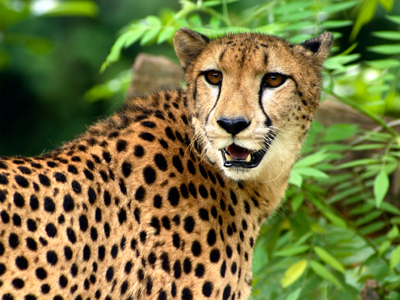A cheetah can run at speeds of up to 120 km/h.

# Speed 03

## Test yourself on speeds on Earth. How fast does a snail move?

### Scroll down to play the quiz

This KS3 Science quiz is our third look at speed. Did you know that the average speed of a common snail is 0.001 m/s (metres per second)? Or that the slowest animal on Earth is the coral - it doesn't actually move!

Speeds on Earth are always given with reference to the Earth itself but the surface of the Earth is moving at a great speed. At the equator, it is moving at a speed of about 1600 km/h as the Earth rotates on its axis. The Earth is also in orbit around the Sun, travelling about 170,000 km every hour. Then you need to consider that the Sun (plus the rest of the Solar System of course) is moving through space as well at about 70,000 km/h in the direction of the star Vega. The Sun is also orbiting the centre of our galaxy at a speed of 800,000 km/h. Finally, our whole galaxy is moving through space at a speed of about 2 million km/h. In one hour, you will have travelled a long way indeed!

Speed always needs a reference point like the surface of the Earth or the centre of our galaxy. It was thinking about speed and reference points that led Albert Einstein to develop his theory of relativity.

1.
If distance is measured in metres and speed in m/s, time is measured in .......
seconds
minutes
hours
None of these
Seconds are the basic SI unit of time and for calculations involving speed, you often need to convert hours into seconds to get the correct answer
2.
How long would it take to run 800 m at a speed of 8 m/s?
80 seconds
100 seconds
1 hour
100 minutes
Time = distance/speed so 800 m / 8 m/s = 100 s
3.
A car travels at 70 mph. How long will it take to travel 210 miles?
20 minutes
2.1 hours
3 hours
7 hours
Time = 210 miles/70 mph = 3 hours
4.
How long would it take to run 400 m at a speed of 5 m/s?
20 seconds
80 seconds
80 minutes
8 hours
Time = distance/speed so 400 m / 5 m/s = 80 s
5.
How long would it take to ride a motorbike 300,000 m at a speed of 60 km/h?
0.5 hours
5 hours
5,000 minutes
5,000 hours
300,000 m = 300 km so time = 300 km / 60 km/h = 5 hours. Light does it in 0.1 seconds!
6.
If distance is measured in kilometres and speed in km/h, time is measured in .......
seconds
minutes
hours
None of these
In many situations, it is easier to understand speeds in terms of km/h rather than m/s
7.
How long would it take to travel 100 km at a speed of 50 km/h?
2 hours
60 minutes
5,000 seconds
5 hours
Time = distance/speed so 100 km / 50 km/h = 2 hours
8.
A cheetah runs at 100 km/h. How long will it take to run 100 metres?
1 second
0.001 hours
1 minute
1 hour
100 m = 0.1 km so time = 0.1/100 = 0.001 hours, 1 hour = 3,600 seconds so 0.001h = 3.6 seconds! The fastest humans take over 9 seconds to cover the same distance
9.
If distance is measured in miles and speed in mph, time is measured in .......
seconds
minutes
hours
None of these
When answering questions about speed, you need to pay close attention to the units and sometimes you will need to convert the units into something more suitable for carrying out the calculation
10.
How long would it take to travel 3 km at a speed of 15 m/s?
5 seconds
200 seconds
45 minutes
2 hours
3 km = 3,000 m so time = 3,000 m / 15 m/s = 200 s